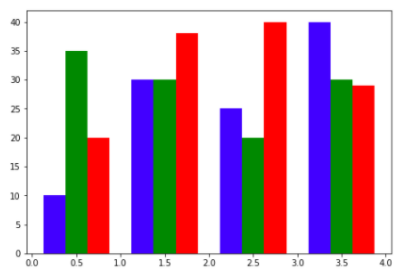# Matplotlib: Compare Values with Different Color in Bar Chart

In this tutorial, we will compare values with different color in one bar chart. You can implement it in matplotlib.1.Import library

```import matplotlib.pyplot as plt
import numpy as np```

2.Prepare compared data

```data = [[10,30,25,40],
[35,30,20,30],
[20,38,40,29]]
X = np.arange(4)```

In this example code, we will compare three group data.

3.Create a bar chart to compare

```fig = plt.figure()
`plt.show()`